# ISEE Mathematics Achievement Practice Test 8

### Test Information15 questions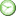15 minutes

Take more free ISEE Mathematics Achievement Practice Tests available from crackssat.com.

1.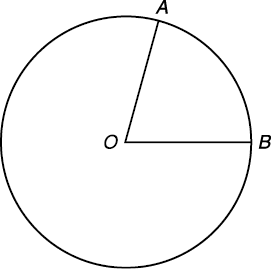In the figure above, m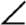AOB = 60°. If O is the center of the circle, then minor arc AB is what part of the circumference of the circle

• A.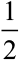• B.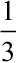• C.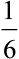• D.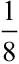2. If all P are S and no S are Q, it necessarily follows that

• A. all Q are S.
• B. all Q are P.
• C. no P are Q.
• D. no S are P.

3. A is older than B. With the passage of time the

• A. ratio of the ages of A and B remains unchanged.
• B. ratio of the ages of A and B increases.
• C. ratio of the ages of A and B decreases.
• D. difference in their ages varies.

4. From a temperature of 15°, a drop of 21° would result in a temperature of

• A. 36°
• B. -6°
• C. -30°
• D. -36°

5.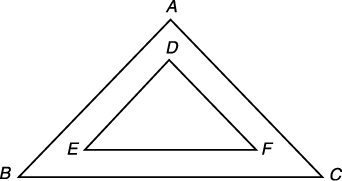In the figure above, the sides of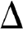ABC are respectively parallel to the sides of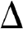DEF If the complement of A is 40°, what is the complement of D

• A. 20°
• B. 40°
• C. 50°
• D. 60°

6. A line of print in a magazine article contains an average of 6 words. There are 5 lines to the inch. If 8 inches are available for an article that contains 270 words, how must the article be changed

• B. Delete 30 words.
• C. Delete 40 words.

7.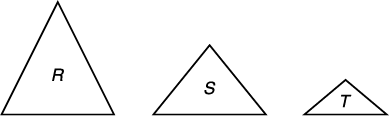The area of triangle R is 3 times triangle S. The area of triangle S is 3 times triangle T. If the area of triangle S = 1, what is the sum of the areas of the three triangles

• A.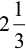• B.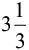• C.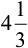• D. 6

8. If 5 pints of water are needed to water each square foot of lawn, the minimum gallons of water needed for a lawn 8' by 12' is

• A. 5
• B. 20
• C. 40
• D. 60

9. In the formula l = p + prt, what does l equal when p = 500, r = 20%, t = 2

• A. 700
• B. 8000
• C. 10,000
• D. 12,000

10. A car owner finds he needs 12 gallons of gas for each 120 miles he drives. If he has his carburetor adjusted, he will need only 80% as much gas. How many miles will 12 gallons of gas then last him

• A. 90
• B. 96
• C. 150
• D. 160

11. What is the maximum number of books, each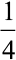-inch thick, that can be placed standing on a shelf that is 4 feet long

• A. 16
• B. 48
• C. 96
• D. 192

12. In a bag there are red, green, black, and white marbles. If there are 6 red, 8 green, 4 black, and 12 white, and one marble is to be selected at random, what is the probability it will be white

• A.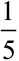• B.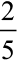• C.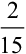• D.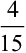13.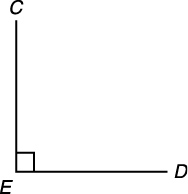In the diagram above,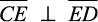. If CE = 7 and ED = 6, what is the shortest distance from C to D

• A. 6
• B. 7
• C.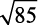• D.14. If a = 3, then aa o a =

• A. 9
• B. 18
• C. 51
• D. 81

15. (3 + 2)(6 - 2)(7 + 1) = (4 + 4)(x). What is the value of x

• A. 13 + 2
• B. 14 + 4
• C. 4 + 15
• D. 8 + 12